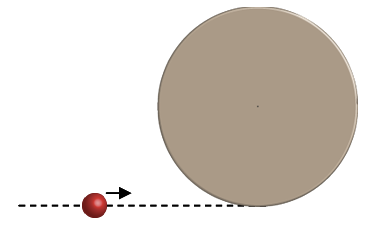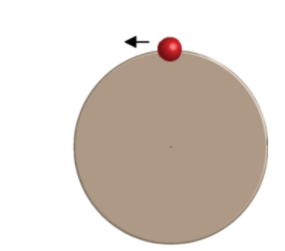# Problem: A merry-go-round with a a radius of R = 1.63 m and moment of inertia I = 196 kg-m2 is spinning with an initial angular speed of ω = 1.53 rad/s in the counter clockwise direection when viewed from above. A person with mass m = 73 kg and velocity v = 4.2 m/s runs on a path tangent to the merry-go-round. Once at the merry-go-round the person jumps on and holds on to the rim of the merry-go-round.1) What is the magnitude of the initial angular momentum of the merry-go-round?2) What is the magnitude of the angular momentum of the person 2 meters before she jumps on the merry-go-round?3) What is the magnitude of the angular momentum of the person just before she jumps on to the merry-go-round?4) What is the angular speed of the merry-go-round after the person jumps on?5) Once the merry-go-round travels at this new angular speed, with what force does the person need to hold on?6)Once the person gets half way around, they decide to simply let go of the merry-go-round to exit the ride.  What is the magnitude of the linear velocity of the person right as they leave the merry-go-round?7) What is the angular speed of the merry-go-round after the person lets go?

###### FREE Expert Solution

This problem involves calculating and conserving angular momentum.

1)

Angular momentum:

$\overline{){\mathbf{L}}{\mathbf{=}}{\mathbf{I}}{\mathbf{\omega }}}$

97% (134 ratings)###### Problem Details

A merry-go-round with a a radius of R = 1.63 m and moment of inertia I = 196 kg-m2 is spinning with an initial angular speed of ω = 1.53 rad/s in the counter clockwise direection when viewed from above. A person with mass m = 73 kg and velocity v = 4.2 m/s runs on a path tangent to the merry-go-round. Once at the merry-go-round the person jumps on and holds on to the rim of the merry-go-round.1) What is the magnitude of the initial angular momentum of the merry-go-round?

2) What is the magnitude of the angular momentum of the person 2 meters before she jumps on the merry-go-round?

3) What is the magnitude of the angular momentum of the person just before she jumps on to the merry-go-round?

4) What is the angular speed of the merry-go-round after the person jumps on?

5) Once the merry-go-round travels at this new angular speed, with what force does the person need to hold on?

6)Once the person gets half way around, they decide to simply let go of the merry-go-round to exit the ride.  What is the magnitude of the linear velocity of the person right as they leave the merry-go-round?

7) What is the angular speed of the merry-go-round after the person lets go?